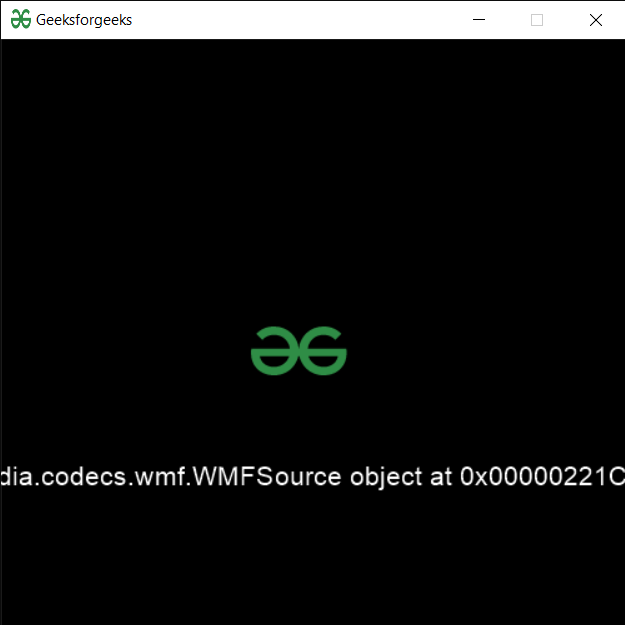In this article, we will see how we can load a media file in PYGLET module in python. Pyglet is easy to use but powerful library for developing visually rich GUI applications like games, multimedia, etc. A window is a “heavyweight” object occupying operating system resources. Windows may appear as floating regions or can be set to fill an entire screen (fullscreen). In order to load a file i.e resource we use resource module of pyglet. This module allows applications to specify a search path for resources. Relative paths are taken to be relative to the application’s __main__ module. Media is basically sound of video resource files

We can create a window object with the help of command given below

```# creating a window
window = pyglet.window.Window(width, height, title)```

In order to do this we use media method with the pyglet.resource
Syntax : resource.media(file_name)
Argument : It takes string i.e file name as argument
Return : It returns media.Source object

Below is the implementation

## Python3

 `# importing pyglet module ` `import` `pyglet ` `import` `pyglet.window.key as key` `  `  `# width of window ` `width ``=` `500` `  `  `# height of window ` `height ``=` `500` `  `  `# caption i.e title of the window ` `title ``=` `"Geeksforgeeks"` `  `  `# creating a window ` `window ``=` `pyglet.window.Window(width, height, title) ` `  `  `# text  ` `text ``=` `"Welcome to GeeksforGeeks"` ` `  `# creating label with following properties` `# font = cooper` `# position = 250, 150` `# anchor position = center` `label ``=` `pyglet.text.Label(text, ` `                          ``font_name ``=``'Cooper'``, ` `                          ``font_size ``=` `16``, ` `                          ``x ``=` `250``,  ` `                          ``y ``=` `150``, ` `                          ``anchor_x ``=``'center'``,  ` `                          ``anchor_y ``=``'center'``)`     `# creating a batch ` `batch ``=` `pyglet.graphics.Batch()`   `# loading geeksforgeeks image` `image ``=` `pyglet.image.load(``'gfg.png'``)`     `# creating sprite object` `# it is instance of an image displayed on-screen` `sprite ``=` `pyglet.sprite.Sprite(image, x ``=` `200``, y ``=` `230``)` `  `  `# on draw event ` `@window``.event ` `def` `on_draw(): ` `      `  `    ``# clear the window ` `    ``window.clear() ` `      `  `    ``# draw the label` `    ``label.draw() ` `    `  `    ``# draw the image on screen` `    ``sprite.draw()` `      `  `# key press event     ` `@window``.event ` `def` `on_key_press(symbol, modifier): ` `  `  `    ``# key "C" get press ` `    ``if` `symbol ``=``=` `key.C: ` `        `  `        ``# printing the message` `        ``print``(``"Key : C is pressed"``)` `        `  `# image for icon ` `img ``=` `image ``=` `pyglet.resource.image(``"gfg.png"``) `   `# setting image as icon ` `window.set_icon(img) `   `# loading media file` `value ``=` `pyglet.resource.media(``"media.mp4"``)`   `# setting text  of label` `label.text ``=` `str``(value)`   `# start running the application ` `pyglet.app.run() `

Output :Whether you're preparing for your first job interview or aiming to upskill in this ever-evolving tech landscape, GeeksforGeeks Courses are your key to success. We provide top-quality content at affordable prices, all geared towards accelerating your growth in a time-bound manner. Join the millions we've already empowered, and we're here to do the same for you. Don't miss out - check it out now!